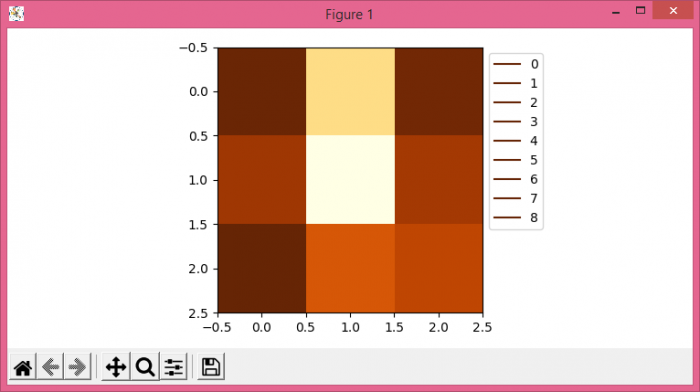# How to add legend to imshow() in Matplotlib?

To add legend to imshow() in Matplotlib, we can take the following steps −

• Set the figure size and adjust the padding between and around the subplots.
• Create random data using numpy.
• Initialize a color map.
• Get the unique data points from sample data, step 2.
• Plot each color with different labels and color, to place on the legend.
• Place a legend at the upper right corner within a box.
• To display the figure, use show() method.

## Example

import numpy as np
from matplotlib import pyplot as plt, cm

plt.rcParams["figure.figsize"] = [7.50, 3.50]
plt.rcParams["figure.autolayout"] = True

data = np.random.rand(3, 3)
cmap = cm.YlOrBr
unique_data = np.unique(data)
i = 0

for entry in unique_data:
mycolor = cmap(entry * 255 / (max(unique_data) - min(unique_data)))
plt.plot(0, 0, "-", color=mycolor, label="%d"%i)
i += 1

plt.imshow(data, cmap=cmap)
plt.legend(loc="upper right", bbox_to_anchor=(1.25, 1.0))

plt.show()

## Output